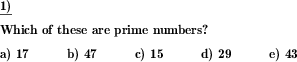Custom math worksheets at your fingertips# Details for problem "Prime test"

Quickname: 6003

Elementary School, Primary School, Junior High School, Middle School.

## Summary

Determine if number is prime.

## Example## Description

A positive natural number is given. A statement has to be made on whether this is a prime number or explain why it’s not.

A very concrete type of problem, when working with prime numbers in fifth-grade or sixth-grade, to be used as a "do now" bell-ringer activity, anticipatory set, closure exercise. This math problem would also be perfect for a quick assessment tool. The level of complexity can be controlled by selecting several problems and the magnitude of numbers used in the worksheet.

By completing this worksheet successfully, the following milestones can be achieved.

• Multiplication and division concepts will be revised.
• Use divisibility rules to solve the problem.
• Strong concepts of factoring given positive numbers will be achieved through practice.
• The practice of these worksheets will also lay the foundation for factoring integers.
• Provide a basis to achieve a perfect level of understanding the math topics like Prime Factoring.

The number of problems and the number range for the number to be worked on can be chosen. Prime test will be done for given numbers. The number range is up to 100000. The answer key will identify a given number as a prime number and if it’s not a prime number, a factor set of a given number is mentioned as proof or explanation.

Download free printable worksheets for this math problem here. The worksheet contains the problems only, the solution sheet includes the answers. Just click on the respective link.

•Worksheet 1Solution sheet with answers
•Worksheet 2Solution sheet with answers
•Worksheet 3Solution sheet with answers

If you can not see the solution sheets for download, they may be filtered out by an ad blocker that you may have installed. If this is the case, please allow ads for this page and reload the page. The solution sheets will then reappear.

• Do these sample worksheets do not really fit?
• Do you need more math worksheets, with a different level of difficulty?
• Would you like to combine different problems on a worksheet and adjust them to your needs?
• As a teacher, you can put together your own worksheets using the automatically generated math problems provided.
With a free initial credit, you can start creating your own math worksheets in a few minutes.

You can try it for free! Register here, to create custom worksheets now!

## Customization options for this problem

Parameter
Possible values
Number of problems
1, 2, 3, 4, 5, 6, 7, 8, 9, 10
Number range
50, 100, 200, 500, 1000, 2000, 5000, 10000
Greatest factor
no limit, 7, 19, 47, 97